### Home > PC > Chapter 2 > Lesson 2.3.4 > Problem2-115

2-115.
1. Simplify the following rational expressions. Homework Help ✎

1.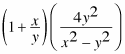2.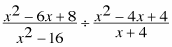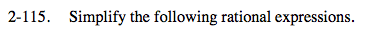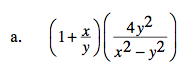Rewrite the first factor as a single fraction. Do this by rewriting 1 as y/y.

$\left(\frac{y+x}{y}\right)\left(\frac{4y^2}{x^2-y^2}\right)$

Factor all expressions and simplify as in problem 2-114.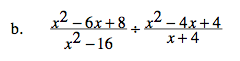Since you are dividing, invert the second fraction and multiply.
Be sure to factor all of the expressions and then simplify.